# 4. A Grammar of Model Analysis: Grama¶

This chapter is about a grammar of model analysis. Just like English grammar, a grammar of model analysis gives rules about how objects fit together; specifically, models, data, and the verbs that relate them. This ruleset is designed to facilitate model analysis under uncertainty.

These rules are implemented in the Python package py-grama. Using the py-grama package, you will be able to reproduce all of the case studies in this book. This means you can immediately take the code in this book and start applying it to your own studies! The py-grama package is free and open source software, hosted on GitHub at this url: https://github.com/zdelrosario/py_grama.

Learning Objectives In this chapter, you will learn

• how to install the py-grama Python package

• key concepts used in py-grama

• the basics of using py-grama to do model analysis under uncertainty, through a quick case study

To best-use this book, you should follow the Quick Start instructions below to install py-grama and get familiar with the software.

## 4.1. Quick Start¶

This quick start guide will take you through installing py-grama, loading in a simple model, and analyzing with some exploratory model analysis tools. The purpose of this quick start is not to solve a scientific problem, but rather to introduce the py-grama toolkit with a very simple model.

### 4.1.1. Install¶

Follow these instructions to install Python and the py-grama package.

#### 4.1.1.1. Install Anaconda Python.¶

Anaconda is a way to install Python that includes many tools for scientific computing. We recommend starting from Anaconda to help take care of dependencies.

#### 4.1.1.2. Use the Python package manager to install py-grama¶

Open your Anaconda prompt, type the following line into your prompt, and hit return (enter).

$pip install py-grama  This will automatically download Grama and install its dependencies. Prompt vs Python Throughout this book, we will use the symbol$ to denote code you should run in your Anaconda prompt. This will be infrequent, but important to notice!

Start a Python terminal (Open Anaconda prompt, and use the command python) and run the code:

import grama as gr


If this runs without error, then you have successfully installed Grama!

### 4.1.2. Jupyter Notebooks¶

Above, you ran Python code in a terminal; this is helpful for using Python like a calculator, but does not allow you to save your work. Python code is sometimes saved in scripts; these are machine-readable files with the .py extension. Scripts are great for reusable code, but we prefer Jupyter notebooks for organizing studies involving equations, figures, and documentation.

Jupyter notebooks are great for writing scientific reports; in fact, the chapters of this book were written as Jupyter notebooks 1!

To start Jupyter, open your anaconda prompt and run the command:

\$ jupyter lab


This will open a page in your web browser with the JupyterLab interface. The JupyterLab documentation has more information; in particular, this overview page has a good introduction to using Jupyter. We highly recommend watching at least an introductory tutorial to learn how to use Jupyter notebooks.

To get the most out of this book, we highly recommend you follow along with this Quick Start! Fire up a Jupyter notebook and try the commands below.

### 4.1.3. Boilerplate code¶

To set up py-grama in a Jupyter notebook, you must run the following code.

### Boilerplate code for setting up py-grama
import grama as gr   # This imports the py-grama package
DF = gr.Intention()  # This sets up the DataFrame "pronoun"


This code loads py-grama and sets up some key infrastructure for the package.

Package prefix

Note that the call to Intention() uses the gr prefix we set with import grama as gr. This is how we access all functions and objects in py-grama, unless we do from grama import something as new_name.

Next, we’ll go through a quick demo of py-grama.

### 4.1.4. A demo model¶

Run the following code to load a built-in py-grama model and print a summary.

from grama.models import make_test
md_test = make_test()
md_test

model: None

inputs:
var_det:
x2: [-1, 1]

var_rand:
x0: (+0) uniform, {'loc': -1, 'scale': 2}
x1: (+0) uniform, {'loc': -1, 'scale': 2}

copula:
Independence copula

functions:
f0: ['x0', 'x1', 'x2'] -> ['y0']


We’ll discuss these elements of a model more below; for now, note that we have three inputs ['x0', 'x1', 'x2'] that map to one output ['y0']. We can get a sense for how the model behaves by constructing a sinew plot, as the following code demonstrates.

(
md_test
>> gr.ev_sinews(df_det="swp")
>> gr.pt_auto()
)

Calling plot_sinew_outputs....

<seaborn.axisgrid.FacetGrid at 0x7fa11cb8fc10>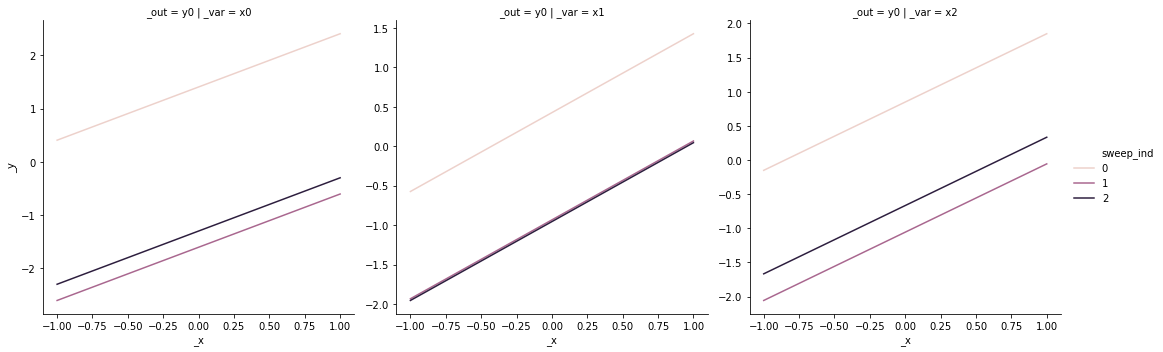Note that the resulting plot has three columns and one row; these correspond to the three inputs (columns and horizontal axes) and one output (rows and vertical axes). Each column of this plot shows a “sweep” along a single input while holding the other inputs constant. Since model behavior can change depending on the held-constant inputs, the process repeats for a variety of starting conditions, resulting in multiple lines in each panel.

All linear?

Interpreting the plot above, it seems that the output 'y0' is linear in all of its inputs: no matter where we start, moving along one of the inputs results in a linear trend for the output. Maybe we got lucky? With more sweeps, would we see different behavior?

### 4.1.5. Overriding the defaults¶

The py-grama package is designed around providing sensible defaults to get you started with each function, but you can override these defaults when necessary. For instance, the following code plots more sweeps by overriding the default n_sweeps = 10.

(
md_test
>> gr.ev_sinews(
df_det="swp",
n_sweeps=10,
)
>> gr.pt_auto()
)

Calling plot_sinew_outputs....

<seaborn.axisgrid.FacetGrid at 0x7fa11f49d2d0>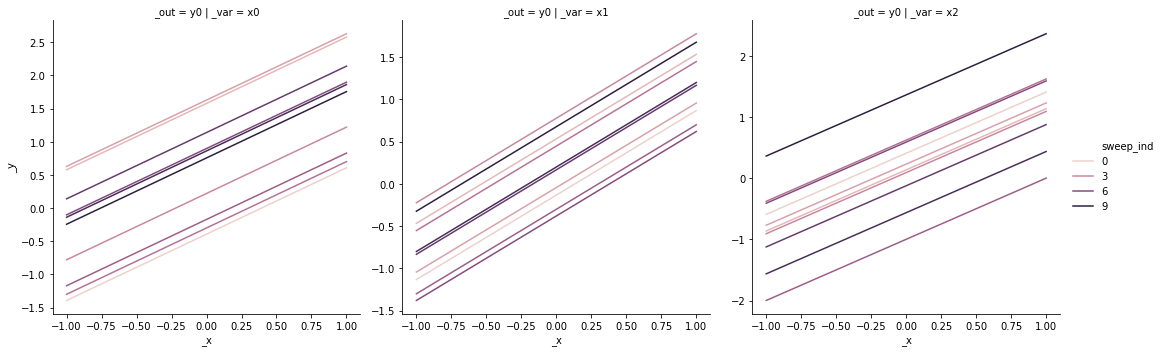Note that more sweeps (larger n_sweeps) will tend to require more compute time 2. This might be easy to afford if your model is simple, but can be quite expensive as your model grows more complicated.

Probably linear

Interpreting the plot above, even with more sweeps the output 'y0' still seems to be linear in all of its inputs. This is hardly proof that 'y0' is linear in all its inputs, but the evidence certainly seems convincing for now.

### 4.1.6. Getting help¶

No programmer memorizes everything in a software package! Competent programmers regularly consult documentation to remind themselves how to use a function, or to learn about a new function.

In Python, you can use the help() command to get more information on a function:

help(gr.eval_sinews)

Help on curry in module grama.eval_random:

eval_sinews(model='__no__default__', n_density=10, n_sweeps=3, seed=None, df_det=None, varname='sweep_var', indname='sweep_ind', append=True, skip=False)
Sweep study

Perform coordinate sweeps over each model random variable ("sinew" design).
Use random starting points drawn from the joint density.

Use gr.plot_auto() to construct a quick visualization of the output
dataframe. Use skip version to visualize the design, and non-skipped
version to visualize the results.

Args:
model (gr.Model): Model to evaluate
n_density (numeric): Number of points along each sweep
n_sweeps (numeric): Number of sweeps per-random variable
seed (int): Random seed to use
df_det (DataFrame): Deterministic levels for evaluation;
use "nom" for nominal deterministic levels,
use "swp" to sweep deterministic variables
varname (str): Column name to give for sweep variable; default="sweep_var"
indname (str): Column name to give for sweep index; default="sweep_ind"
append (bool): Append results to conservative inputs?
skip (bool): Skip evaluation of the functions?

Returns:
DataFrame: Results of evaluation or unevaluated design

Examples:

>>> import grama as gr
>>> md = gr.make_cantilever_beam()
>>> # Skip evaluation, vis. design
>>> df_design = md >> gr.ev_sinews(df_det="nom", skip=True)
>>> df_design >> gr.pt_auto()
>>> # Vis results
>>> df_sinew = md >> gr.ev_sinews(df_det="nom")
>>> df_sinew >> gr.pt_auto()


Reading a function’s documentation can be overwhelming at first, but with practice you can quickly find a lot of useful information:

• The line with eval_sinews(...) is the function signature; this shows the function with all of its possible arguments.

• The paragraphs that follow the signature describe the function in plain language.

• The lines under Args: detail the arguments to the function. The lines are of the form name (type): Details, where

• name is the name of the argument

• type is the expected datatype of the argument

• Details follow in plain-text. If an argument only takes particular values, the Details should tell you about this

• The line under Returns: details the returned object (if any), including its datatype.

• Function documentation often includes Examples:; look at this section for some real-code examples of the function’s use. This is particularly useful, as you can see what other objects and functions this tool is used with.

Documentation in Jupyter

Note that in Jupyter, you can quickly reference the documentation for a function by placing your cursor over a function you’d like to reference, and pressing the keys Shift + Tab. Try this in your own Jupyter notebook!

## 4.2. Grama Concepts¶

Now that we’ve seen how py-grama works, let’s look closer at the concepts behind py-grama. The py-grama package was designed around a few simple ideas: two primary kinds of objects (data and models) and four kinds of verbs.

### 4.2.1. Objects: Data and Models¶

Data (df)

Data in py-grama is organized into Pandas DataFrames. A DataFrame is a digital table, with named columns that organize observations on variables. It is a convention when using py-grama to name your DataFrames with the df_ prefix followed by a descriptive name, for instance df_train for a set of training data.

The code we saw above generated a DataFrame, which was then visualized using the gr.pt_auto() tool. If we disable the plotting line with a comment, we can inspect the data.

df_results = (
md_test
>> gr.ev_sinews(df_det="swp")
#     >> gr.pt_auto()
)
df_results


x0 x1 x2 sweep_var sweep_ind y0
0 -1.000000 -0.444582 -0.342595 x0 0 -1.787177
1 -0.777778 -0.444582 -0.342595 x0 0 -1.564954
2 -0.555556 -0.444582 -0.342595 x0 0 -1.342732
3 -0.333333 -0.444582 -0.342595 x0 0 -1.120510
4 -0.111111 -0.444582 -0.342595 x0 0 -0.898288
... ... ... ... ... ... ...
85 -0.791376 -0.501520 0.111111 x2 2 -1.181785
86 -0.791376 -0.501520 0.333333 x2 2 -0.959563
87 -0.791376 -0.501520 0.555556 x2 2 -0.737340
88 -0.791376 -0.501520 0.777778 x2 2 -0.515118
89 -0.791376 -0.501520 1.000000 x2 2 -0.292896

90 rows × 6 columns

Note that the DataFrame df_results contains both numeric columns (such as df_results.x1) and string columns (such as df_results.sweep_var). We can also see some basic-but-useful facts, such as the size (90 rows x 6 columns).

On their own, data are not immediately useful. We will see a variety of transformation verbs we can use in py-grama to work with data, and we will use data to inform and even fit models.

The data we work with can come from a physical experiment (loaded from disk) or from a simulation (generated by a model). Either way, it will be packaged in a Pandas DataFrame. This ensures we can use consistent tools to work with data, regardless of the origin.

Models (md)

Models in py-grama are represented by a special gr.Model Python class. A model is essentially a capsule containing functions that map inputs to outputs, and information about the inputs. We saw some of this information summarized when we printed md_test above.

md_test

model: None

inputs:
var_det:
x2: [-1, 1]

var_rand:
x0: (+0) uniform, {'loc': -1, 'scale': 2}
x1: (+0) uniform, {'loc': -1, 'scale': 2}

copula:
Independence copula

functions:
f0: ['x0', 'x1', 'x2'] -> ['y0']


Reading these lines, we can see the key information about the model summarized as text:

• The inputs organize the model inputs into two categories:

• var_det are the deterministic variables; these are assumed to be perfectly known, and must be set in order to run the model. Deterministic variables are reported with their bounds

• var_rand are the random variables; these are assumed to be fundamentally unknowable, and can be sampled according to their distribution. Random variables are reported with their distribution type and parameters.

• The copula describes the relationship between random variables. In the example above, the random variables are assumed to be mutually independent.

• The functions list all of the mappings from inputs to outputs.

• The example above has just one function f0, which maps all of its inputs to the single output y0.

In py-grama, models are used to represent scenarios with quantified uncertainties. We can use models to run simulations (generate synthetic data), to propagate uncertainty, or even as a template to fit a model to data.

### 4.2.2. Verbs: Four fundamental verbs¶

The py-grama package focuses on data and model objects, and provides four kinds of verbs that inter-relate the two object types. The relationship between these objects and verbs constitutes the grammar of this grammar of model analysis.

In short, the four verbs relate data and models according to Figure Fig. 4.1.Fig. 4.1 The py-grama verbs, as they relate to data and models.

In greater detail, the four verbs are:

• A transformation (tran_) transforms one DataFrame into another DataFrame. We might want a transformation to perform some simple calculation (like converting from Fahrenheit to Celsius), to reshape a dataset, to convert from one datatype to another, and so on.

• An evaluation (eval_) uses a Model to generate a DataFrame. Evaluation routines are used to run a variety of simulations, such as a simple planned run (gr.eval_df()), a Monte Carlo simulation (gr.eval_monte_carlo()), or a more advanced reliability-assessment technique (e.g. gr.eval_form_pma()).

• A fit (fit_) uses a training DataFrame to generate a Model. We could start with a user-defined model and fit its free parameters (gr.fit_nls()), or use a purely empirical approach to modeling the data (e.g. gr.fit_gp()).

• A composition (comp_) is used to modify an existing Model. Composition routines are generally used in the model-building phase of a study, though we can also use them to make small adjustments to a model.

Like any language, there are also some exceptional verbs:

• Plotting routines (plot_) are used to visualize data. You are of course free to plot your data however you like, but know that the magic gr.plot_auto() routine is available to provide a quick visualization for many of the outputs of eval_ verbs.

The following table summarizes the verbs in terms of their prefixes and input-output datatypes.

Table 4.1 Table of verbs and prefixes.

Verb

Short

In

Out

tran_

tf_

df

df

eval_

ev_

md

df

fit_

ft_

df

md

comp_

cp_

md

md

plot_

pt_

df

Recognizing a new verb

The verb prefixes tran_ (tf_), eval_ (ev_), fit_ (ft_), and comp_ (cp_) are signifiers to help you recognize something about a verb just by looking at it. Remember that the prefix will tell you the type of the verb’s first input, and the type of its return value.

### 4.2.3. Functional programming¶

The py-grama package also provides some special syntax for functional programming-style computational pipelines. One of the advantages of this style of programming is that we can eliminate intermediate variable names. For instance, consider the following imperative-style code:

## Build the model
md_temporary = gr.Model("Temporary model")
md_temporary = gr.comp_function(
md_temporary,
fun=lambda X: X * X,
var=2,
out=1
)
md_temporary = gr.comp_bounds(
md_temporary,
x0=(0, 1),
x1=(0, 1),
)

## Generate results
df_temporary = gr.eval_sinews(
md_temporary,
df_det="swp",
)

## Visualize
gr.plot_auto(df_temporary)

Calling plot_sinew_outputs....

<seaborn.axisgrid.FacetGrid at 0x7fa11e25b850>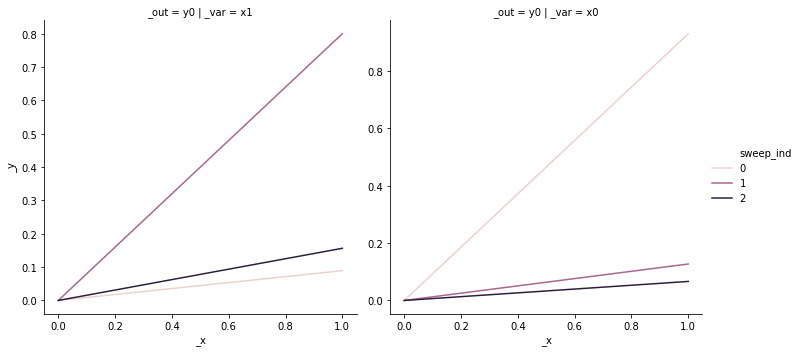Now compare with functional-style code:

(
gr.Model("Temporary model")
>> gr.cp_function(
fun=lambda X: X * X,
var=2,
out=1,
)
>> gr.cp_bounds(
x0=(0, 1),
x1=(0, 1),
)
>> gr.ev_sinews(df_det="swp")
>> gr.pt_auto()
)

Calling plot_sinew_outputs....

<seaborn.axisgrid.FacetGrid at 0x7fa11e5f8ad0>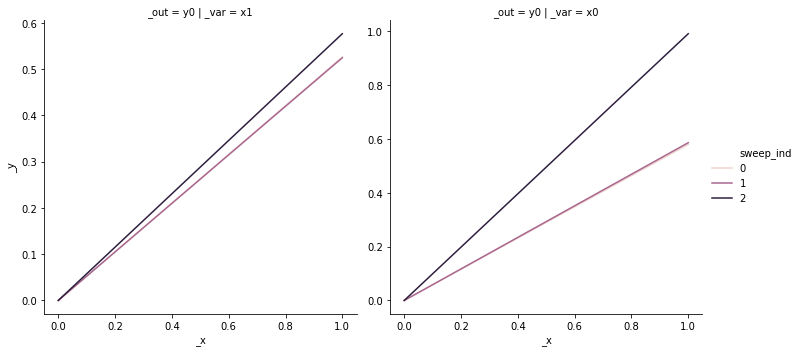The functional-style code is more compact and does not require us to set intermediate variable names. However, it can be confusing to read—at first.

One idea that helps make functional-style code more readable is to think of the pipe operator >> as the phrase “and then”. This makes the following code:

(
gr.Model(...)
>> gr.cp_function(...)
>> gr.cp_bounds(...)
>> gr.ev_sinews(...)
)


(
Create a model
and then compose with a function
and then compose with bounds
and then evaluate sinews
)


Short-prefixes for functional-style code

Note also that the short two-letter prefixes (e.g. ev_) are used for functional-style code, as opposed to the long forms of the prefix (e.g. eval_). If you find that your code throws errors, make sure you’re using the correct form of the prefix!

### 4.2.4. More on py-grama¶

This concludes the brief tour of py-grama. For more information, you can read the Grama Reference appendix entry, and the official documentation, hosted at https://py-grama.readthedocs.io/en/latest/.

## 4.3. A Brief Case Study: Assessing Structural Safety¶

We end this chapter with an example of using py-grama to analyze the safety of a structural plate, subject to compressive forces. Plates are common in many kinds of structures; for instance, the skin of an airplane wing is designed to take some of the bending loads due to lift generation. If one of these skin panels buckles, it will crumple and potentially lead to unsafe deformation of the wing. However, variability in manufacturing means no two plates will perform exactly the same. We will analyze the safety of plates under buckling by performing a reliability analysis—assessing the probability of failure.

All scientific and engineering projects should have some sort of goal; the case studies in this book are no different.

Goals for this case study

In studying these plates, our goal is to determine an appropriate thickness of plate that will support a desired compressive load of 1 ksi with a low chance of buckling. For this study, we seek a failure rate less than 1 in a 100.

First, we set up the case study by importing some data on aluminum plate material properties, and load a package to visualize the data.

import plotnine as pt           # For visualizing data
from grama.data import df_stang # Dataset for modeling


Install plotnine

If you do not have plotnine installed, you can install it from Anaconda prompt with the command pip install plotnine.

Representative data

In order for data to be appropriate for our study, they must be representative of the manufacturing process we will use to produce the plates. (It can’t just be any old data!) In this case study, we will assume the data come from our desired manufacturing process.

Let’s inspect the data we just loaded.

df_stang

thick alloy E mu ang
0 0.022 al_24st 10600 0.321 0
1 0.022 al_24st 10600 0.323 0
2 0.032 al_24st 10400 0.329 0
3 0.032 al_24st 10300 0.319 0
4 0.064 al_24st 10500 0.323 0
... ... ... ... ... ...
71 0.064 al_24st 10400 0.327 90
72 0.064 al_24st 10500 0.320 90
73 0.081 al_24st 9900 0.314 90
74 0.081 al_24st 10000 0.316 90
75 0.081 al_24st 9900 0.314 90

76 rows × 5 columns

These are observations on the elasticity E (ksi) and Poisson ratio mu (-) of a rolled aluminum alloy, with multiple specimens prepared and tested [SGN46]. Elasticity is a measure of “stiffness” of a material 3; the higher the E value, the more force it takes to stretch a part. The Poisson ratio is sometimes called the volume contraction ratio; by way of example, when stretching a rubber band, the band will tend to get thinner in the middle. The Poisson ratio of a material determines how much thinner a part will tend to get when stretched. These two properties affect the buckling strength of a plate; we’ll see this when we study the buckling equation below.

However, before we study buckling, we will use these data to build a model for the uncertainty in the material properties.

### 4.3.1. Building the model¶

When building a model from data, we should first inspect the data to make sure the data are trustworthy. This process will help us check our assumptions and data quality.

Properties are thickness-independent?

Material properties such as elasticity and the Poisson ratio are generally thought to be thickness-independent. If this is the case, then we don’t need to worry too much about relating the material properties to the chosen plate thickness, which will greatly simplify modeling. However, we should check this assumption using our data!

The following plot shows Poisson ratio against elasticity, with the thickness of each specimen denoted by the shapes of the points.

(
df_stang
>> gr.tf_mutate(thick=gr.as_factor(DF.thick))
>> pt.ggplot(pt.aes("E", "mu", shape="thick"))
+ pt.geom_point(size=2)
+ pt.theme_minimal()
+ pt.theme(figure_size=(4, 3))
)

Warning: is_categorical is deprecated and will be removed in a future version.  Use is_categorical_dtype instead
Warning: Using shapes for an ordinal variable is not advised.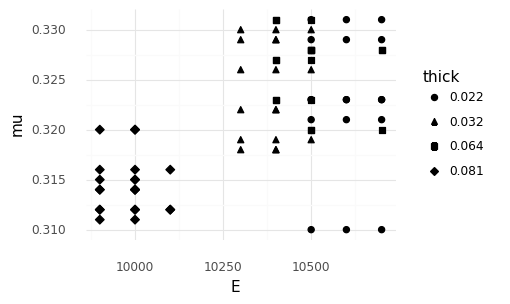<ggplot: (8770623694533)>


This plot implies that the material properties are not thickness independent! However, the patterns in the data are rather suspicious….

Outliers

Based on the figure above, the specimens of thickness 0.081 (in) are suspicious; these observations are well-separated from all the other cases. Reviewing the original publication that reported these data [SGN46], it turns out that the thickest specimens were characterized with a different measurement procedure than the thinner specimens. This suggests that the measurements of the thickest specimens might not be compatible with the measurements of the thinnest specimens.

Let’s filter out the thickest specimens and see if the remaining observations are relatively consistent.

df_filtered = (
df_stang
>> gr.tf_filter(DF.thick < 0.081)
)

(
df_filtered
>> gr.tf_mutate(thick=gr.as_factor(DF.thick))
>> pt.ggplot(pt.aes("E", "mu", shape="thick"))
+ pt.geom_point(size=2)
+ pt.theme_minimal()
+ pt.theme(figure_size=(4, 3))
)

Warning: is_categorical is deprecated and will be removed in a future version.  Use is_categorical_dtype instead
Warning: Using shapes for an ordinal variable is not advised.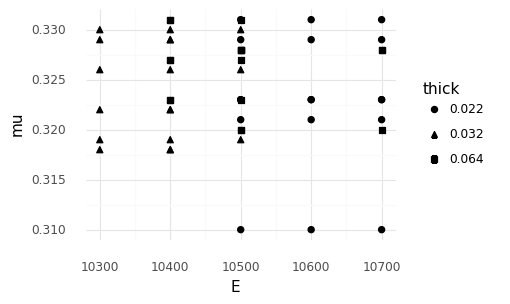<ggplot: (8770626969337)>


The remaining observations are fairly consistent, even across different thicknesses. Given what we know, we will advance by ignoring the thickest specimen data, and limit our attention to plates studied in the remaining data. This leaves us with plates roughly between 1/48” and 1/16” in thickness.

Talk to experts!

If we were in the business of manufacturing plates, this would be an excellent time to go have a chat with the materials and process (M&P) engineers about their experiments. We would ask about the two different characterization procedures, and ask for their opinion on how to use the data to inform our structural reliability analysis. We assumed the thickest specimens were less trustworthy, but their expert opinion might be different.

Now that we have a subset of data that we trust, we can proceed to build a probabilistic model for the uncertainty in material properties. As we’ll see in Chapter TODO, we will need a model (rather than just data) in order to study the very small failure probabilities that we seek. The following code fits a joint distribution for E, mu based on the filtered data.

md_aluminum = (
gr.Model("Plate aluminum material properties")
>> gr.cp_marginals(
E=gr.marg_named(df_filtered.E, "lognorm"),
mu=gr.marg_named(df_filtered.mu, "beta"),
)
>> gr.cp_copula_gaussian(
df_data=df_filtered
)
)
md_aluminum

model: Plate aluminum material properties

inputs:
var_det:

var_rand:
E: (+0) lognorm, {'s': 7.36048659652516, 'loc': 10299.999999999998, 'scale': 4.90067731859436}
mu: (+0) beta, {'a': 6.906673364779303, 'b': 0.6456702700075123, 'loc': 0.2616870498769197, 'scale': 0.06931295012308032}

copula:
Gaussian copula with correlations:
var1 var2      corr
0   mu    E  0.103391

functions:


A reasonable fit?

We chose a couple of marginal distribution shapes above: lognormal for E and beta for mu. How well do these choices represent the original data?

To assess the model fit, visualize the original Experimental data against Synthetic realizations from our distribution model.

(
md_aluminum
## Draw realizations from distribution
>> gr.ev_monte_carlo(n=1e3, seed=101, df_det="nom", skip=True)
>> gr.tf_mutate(source="Synthetic")
## Add in the experimental data
>> gr.tf_bind_rows(
df_filtered
>> gr.tf_mutate(source="Experimental")
)
## Compare the synthetic and experimental data
>> pt.ggplot(pt.aes("E", "mu", color="source"))
+ pt.geom_point()
+ pt.scale_color_manual(
values=dict(Experimental="black", Synthetic="grey"),
name="Source",
)
+ pt.theme_minimal()
+ pt.theme(figure_size=(4, 3))
+ pt.labs(x="Elasticity", y="Poisson ratio", title="Model V1")
)

eval_monte_carlo() is rounding n...
Design runtime estimates unavailable; model has no timing data.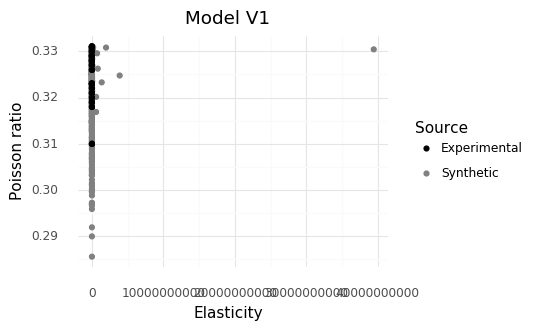<ggplot: (8770623783093)>


Extreme outliers in elasticity!

The chosen shape for the elasticity E produes extreme outliers that do not appear in the original data. We have no evidence to support such extreme tail behavior. We should change the marginal for E.

Update the model to use a marginal shape for E with less extreme tail behavior. Let’s repeat the comparison to check our updated model.

md_aluminum_v2 = (
gr.Model("Plate aluminum material properties")
>> gr.cp_marginals(
E=gr.marg_named(df_filtered.E, "norm"),
mu=gr.marg_named(df_filtered.mu, "beta"),
)
>> gr.cp_copula_gaussian(
df_data=df_filtered
)
)

(
md_aluminum_v2
## Draw realizations from distribution
>> gr.ev_monte_carlo(n=1e3, seed=101, df_det="nom", skip=True)
>> gr.tf_mutate(source="Synthetic")
## Add in the experimental data
>> gr.tf_bind_rows(
df_filtered
>> gr.tf_mutate(source="Experimental")
)
## Compare the synthetic and experimental data
>> pt.ggplot(pt.aes("E", "mu", color="source"))
+ pt.geom_point()
+ pt.scale_color_manual(
values=dict(Experimental="black", Synthetic="grey"),
name="Source",
)
+ pt.theme_minimal()
+ pt.theme(figure_size=(4, 3))
+ pt.labs(x="Elasticity", y="Poisson ratio", title="Model V2")
)

eval_monte_carlo() is rounding n...
Design runtime estimates unavailable; model has no timing data.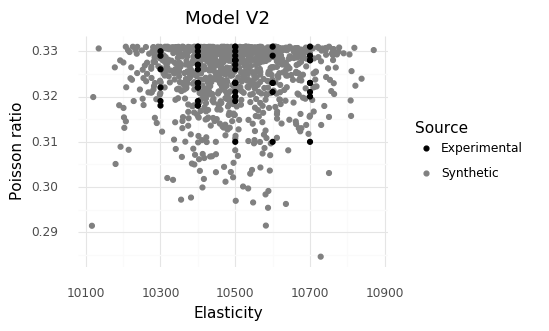<ggplot: (8770627030725)>


This looks much more reasonable! The Synthetic and Experimental data exhibit much more similar variability.

“Gridded” experimental values…

Note that the experimental values seem to lie on a grid: It appears the elasticity values have been rounded to the nearest hundred ksi. The standard deviation of the observed values is around 123 ksi, while the standard deviation of the rounding (assuming a uniform distribution) is $$100 / \sqrt{12} \approx 29$$. This means we are under-accounting for the variability in the data by a small amount. For our purposes this is not likely to cause a severe issue, but for more precise calculations—say, a more strict reliability requirement—this is a factor worth considering.

Now that we have a reasonable model for the material properties, we can turn our attention to mapping these inputs to the buckling behavior of the plate. Buckling is analyzed in terms of the critical buckling stress $$\sigma$$ of a particular part. The larger this stress, the greater capacity the part has to withstand a buckling load.

The critical buckling stress for a plate in compression is a classic result from structural mechanics [Pee11], given by

$\sigma_{\text{buckling}} = \frac{\pi^2 E}{12(1 - \mu^2)}\left(\frac{t}{a}\right)^2,$

with symbols disambiguated in the table below.

Symbol

Meaning

$$E$$

Aluminum elasticity

$$\mu$$

Aluminum Poisson ratio

$$t$$

Plate thickness

$$a$$

Plate length

$$\sigma_{\text{buckling}}$$

Buckling stress

Based on the equation above—and based on general physical intuition—we should have some expectations about the output’s behavior with its inputs. For instance, we would expect the buckling stress to get larger with a larger thickness: a thicker plate is harder to buckle. Conversely, we would expect a longer plate to buckle more easily, leading to a smaller buckling stress.

We can implement this function and add it to our py-grama model. The code below does this.

md_plate = (
md_aluminum_v2
>> gr.cp_vec_function(
fun=lambda df: gr.df_make(
sigma_buckle=3.14159**2 * df.E
/ 12 / (1 - df.mu**2)
* df.a**2 / df.t**2
),
var=["E", "mu", "a", "t"],
out=["sigma_buckle"],
name="Buckling strength",
)
>> gr.cp_bounds(
a=(2, 6),
t=(1/48, 1/16),
)
)
md_plate

model: Plate aluminum material properties

inputs:
var_det:
t: [0.020833333333333332, 0.0625]
a: [2, 6]

var_rand:
E: (+0) norm, {'loc': 10494.444444444445, 'scale': 122.34841969747357}
mu: (+0) beta, {'a': 6.906673364779303, 'b': 0.6456702700075123, 'loc': 0.2616870498769197, 'scale': 0.06931295012308032}

copula:
Gaussian copula with correlations:
var1 var2      corr
0   mu    E  0.030156

functions:
Buckling strength: ['E', 'mu', 'a', 't'] -> ['sigma_buckle']


When implementing a model, it is a good idea to use sinews as a sanity-check. We do so below.

(
md_plate
>> gr.ev_sinews(df_det="swp")
>> gr.pt_auto()
)

Calling plot_sinew_outputs....

<seaborn.axisgrid.FacetGrid at 0x7fa121a0d6d0>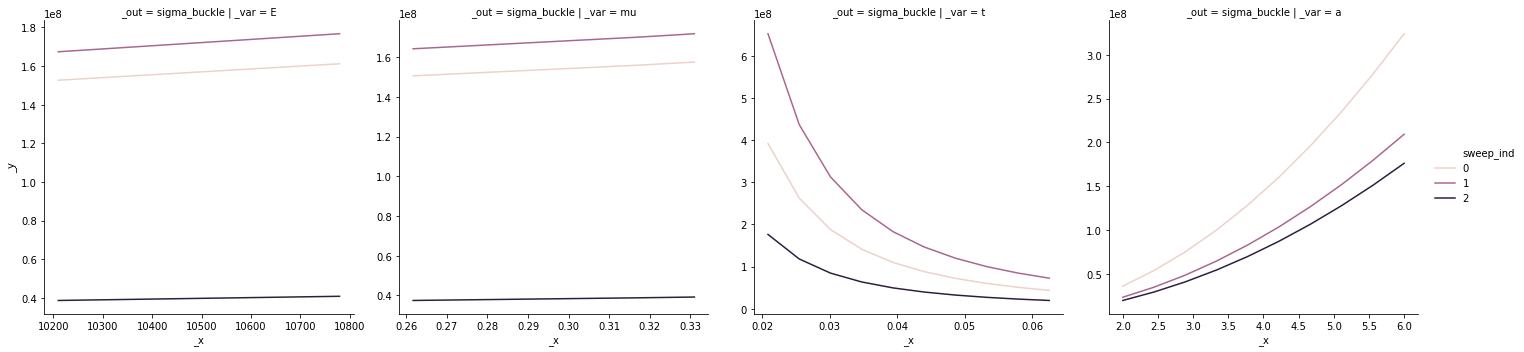Sinews indicate an incorrect model!

This sinew plot doesn’t match the intuition we stated above! As we increase the length a the plate becomes harder to buckle (the buckling stress increases), while when we make the plate thicker the plate becomes easier to buckle (buckling stress decreases).

By sanity-checking the model with a sinew plot, we can see that the model has incorrect behavior. Reviewing the code above, it seems the $$a$$ and $$t$$ terms are swapped. We can fix that easily and re-run the sweeps.

md_plate_v2 = (
md_aluminum_v2
>> gr.cp_vec_function(
fun=lambda df: gr.df_make(
sigma_buckle=3.14159**2 * df.E
/ 12 / (1 - df.mu**2)
* df.t**2 / df.a**2
),
var=["E", "mu", "a", "t"],
out=["sigma_buckle"],
name="Buckling strength",
)
>> gr.cp_bounds(
a=(2, 6),
t=(1/48, 1/16),
)
)

(
md_plate_v2
>> gr.ev_sinews(df_det="swp")
>> gr.pt_auto()
)

Calling plot_sinew_outputs....

<seaborn.axisgrid.FacetGrid at 0x7fa11f1c6090>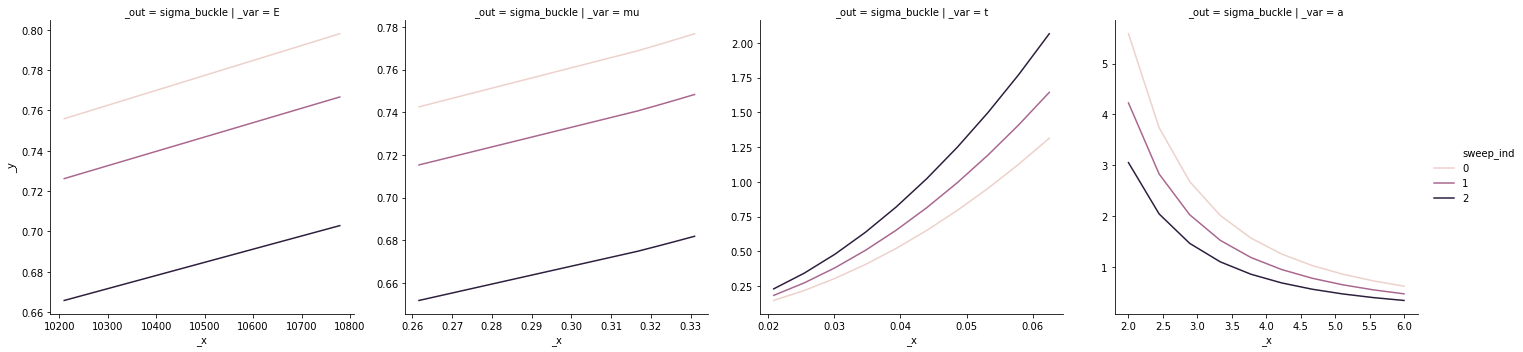This matches our intuition, and provides a quantitative comparison between the inputs. Note that the range of critical buckling values is quite small in the material property panels, while the ranges associated with the plate thickness and length are much wider. This implies that we can adjust the plate dimensions to achieve our desired safety goal, as the buckling behavior is much more sensitive to the plate geometry than material properties.

Unstated part of the goal

Note that we had to specify bounds for the length of the plate to run the sweep. We didn’t specify a plate length above in our goal! To correct this oversight, let’s update our goal to specify plates $$6$$ inches in length.

Finally, to complete our modeling, we will incorporate the target load of $$1$$ ksi by defining a limit state function [DM05]

$g_{\text{buckle}} = \sigma_{\text{buckle}} - \sigma_{\text{applied}},$

with $$\sigma_{\text{applied}} = 1$$ ksi. The limit state function $$g$$ standardizes the interpretation of our quantitative failure model: Cases where $$g > 0$$ correspond to safe operation, while $$g \leq 0$$ correspond to failure.

md_buckling = (
md_plate_v2
>> gr.cp_vec_function(
fun=lambda df: gr.df_make(
g_buckle=df.sigma_buckle - 1.0
),
var=["sigma_buckle"],
out=["g_buckle"],
name="Limit state",
)
)
md_buckling

model: Plate aluminum material properties

inputs:
var_det:
t: [0.020833333333333332, 0.0625]
a: [2, 6]

var_rand:
E: (+0) norm, {'loc': 10494.444444444445, 'scale': 122.34841969747357}
mu: (+0) beta, {'a': 6.906673364779303, 'b': 0.6456702700075123, 'loc': 0.2616870498769197, 'scale': 0.06931295012308032}

copula:
Gaussian copula with correlations:
var1 var2      corr
0   mu    E  0.030156

functions:
Buckling strength: ['E', 'mu', 'a', 't'] -> ['sigma_buckle']
Limit state: ['sigma_buckle'] -> ['g_buckle']


Now we have a complete model with which to conduct our analysis.

### 4.3.2. Analyzing the model¶

We are interested in determining a plate thickness that gives a chance of failure less than 1 in 100. To estimate the probability of failure (POF), we will use a Monte Carlo simulation technique (Chapter TODO) with the probabilistic model we just constructed.

To start, we will analyze a plate with thickness $$1/48$$”; the lower end of sizes considered in our dataset.

(
md_buckling
>> gr.ev_monte_carlo(
n=1e2,
seed=101,
df_det=gr.df_make(a=6, t=1/48),
)
>> gr.tf_summarize(
pof_lo=gr.binomial_ci(DF.g_buckle <= 0, side="lo"),
pof_est=gr.mean(DF.g_buckle <= 0),
pof_up=gr.binomial_ci(DF.g_buckle <= 0, side="up"),
)
)

eval_monte_carlo() is rounding n...

pof_lo pof_est pof_up
0 0.963007 1.0 1.0

Clearly, the probability of failure is much higher than our desired $$0.01$$! Let’s try the other end of the range with a thickness of $$1/16$$”.

(
md_buckling
>> gr.ev_monte_carlo(
n=1e2,
seed=101,
df_det=gr.df_make(a=6, t=1/16)
)
>> gr.tf_summarize(
pof_lo=gr.binomial_ci(DF.g_buckle <= 0, side="lo"),
pof_est=gr.mean(DF.g_buckle <= 0),
pof_up=gr.binomial_ci(DF.g_buckle <= 0, side="up"),
)
)

eval_monte_carlo() is rounding n...

pof_lo pof_est pof_up
0 0.0 0.0 0.036993

The estimated probability of failure pof_est is zero! That certainly satisfies our goal of pof < 0.01, but it seems fishy….

Calculation tolerances

The Monte Carlo approach gives us an estimate for our target quantity, but also a confidence interval (CI). A CI gives us a possible range where the true value may land. The CI we see above violates our constraint (pof_up > 0.01), which indicates our simulation needs more refinement to provide a more trustworthy answer.

We can refine our simulation by increasing the sample size n in our Monte Carlo evaluation. A rough rule-of-thumb when estimating low-probability events is to use a sample size of $$n = 20 / p$$, where $$p$$ is our best-guess for the small probability. Since we’re targeting a failure rate of 1 in 100, we’ll set $$p = 0.01$$ and apply the heuristic.

(
md_buckling
>> gr.ev_monte_carlo(
n=20 / 0.01,
seed=101,
df_det=gr.df_make(a=6, t=1/16)
)
>> gr.tf_summarize(
pof_lo=gr.binomial_ci(DF.g_buckle <= 0, side="lo"),
pof_est=gr.mean(DF.g_buckle <= 0),
pof_up=gr.binomial_ci(DF.g_buckle <= 0, side="up"),
)
)

eval_monte_carlo() is rounding n...

pof_lo pof_est pof_up
0 8.673617e-19 0.0 0.009512

Success! We have refined our results and found a thickness that confidently satisfies our POF constraint. This achieves our goal, and finishes the case study. $$\square.$$

Going further

We found a thickness that satisfies our POF constraint, but we could go further by seeking an optimal thickness that meets the same constraint. In Chapter TODO we will see a more detailed formulation that uses the reliability properties we estimated here within a design optimization.

## 4.4. A general process¶

The brief case study above suggests an interative process you can use to approach model analysis under uncertainty:

• This might include making your goal more specific, as we did with specifying a plate length above.

• Iteratively build a model for your study. This includes specifying the functions, the inputs, and a description of the inputs with bounds or a joint density.

• The ideal approach is to use representative data to inform your model. We used a dataset of measured aluminum properties above.

• If data are not available, consulting literature is an acceptable way to build your model. We used a classic reference to arrive at the buckling function above.

• You will likely discover surprises along the way, as we saw with the thickest plate specimens above. These may necessitate follow-up studies or reasonable assumptions.

• Iteratively analyze your model to provide a trustworthy results that support your goal.

• You will likely have to iterate on your methods to arrive at trustworthy results, as we saw with refining the Monte Carlo simulation above.

Throughout the whole process, use the dual modes of curiosity and skepticism to guide your process.

1

We are thankful to the folks at the Jupyter Book project, whose work enabled this Python-powered textbook!

2

Formally, the computational complexity is linear in n_sweeps.

3

More formally, elasticity is a material property while stiffness is a property of a structure. Elasticity is thought to be a material property, while stiffness is a function of both the material elastic properties, and the geometry of the structure being studied.